Home > CC1 > Chapter 8 > Lesson 8.3.1 > Problem8-91

8-91.
1. The ice cream consumption in several countries is given in the table at right. 8-91 HW eTool (Desmos). Homework Help ✎

1. How many countries are represented?

2. Display the data in a histogram. Make the bins 4 liters per person wide (0-4, 4-8, etc.).

3. Describe the shape of the distribution.

4. Why is it appropriate to calculate a mean for this data? Calculate the mean.

5. Measure the spread (variability) of the data by finding the mean absolute deviation.

6. In complete sentences, completely describe the distribution of ice-cream consumption by discussing the center, shape, spread, and outliers.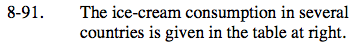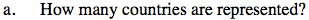Count how many countries there are in the list of countries.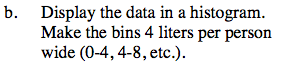Make a histogram with the x-axis scaled by 4s and the
y-axis scaled by 1s. Remember that the y-axis will represent the number of countries that have ice-cream consumptions within each bin.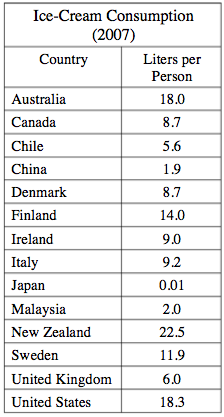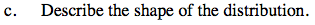Look for symmetry in the graph. Are there any outliers or unusual looking bars in the histogram?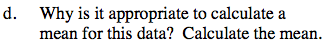Use your answer from part (c) to help with your reasoning for using the mean. Was the data roughly symmetric?

9.7 liters per person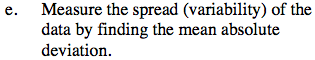Remember that you can calculate the mean absolute deviation by finding the distance each point is from the mean and averaging those distances.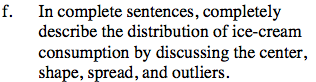Use your previous answers to describe the shape, center, spread, and outliers. Is the histogram symmetrical? Are there any countries that are unusual in their ice-cream consumption? Don't forget to mention the variability of the distribution that you found by calculating the mean absolute deviation!

Use the eTool below to make a histogram to represent the data.
Click the link at right for the full version of the eTool: CC1 8-91 HW eTool